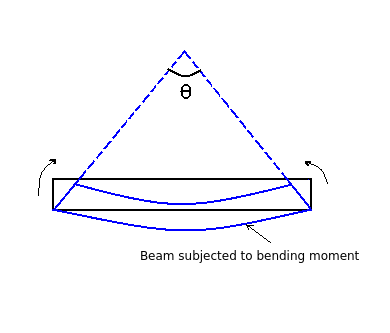# Formula - Strain energy - simply supported beam due to bending moment

Q.  What is the strain energy stored in a simply supported beam due to bending moment?
- Published on 21 Sep 15

a. ∫ (M2/EI)
b. ∫ (M2/2EI)
c. ∫ (M/2EI)
d. ∫ (2M/EI)

#### Discussion

• Sravanthi   -Posted on 24 Nov 15- When a beam is subjected to bending moment, it deflects by an angle θ as shown above.

- Therefore, workdone = (1 / 2) M dθ

But strain energy = workdone

Since, d2y / dx2 = M / EI = dθ / dx

dθ = (M / EI) dx ---------- (2)

Substituting the value of dθ in eq (1),

strain energy = (1 / 2) M dθ

= (1 / 2) M x (M / EI) dx

Strain energy = ∫ [M2 / 2EI] dx

## ➨ Post your comment / Share knowledgeEnter the code shown above:

(Note: If you cannot read the numbers in the above image, reload the page to generate a new one.)